# Java program to find Voting Age Program in Java

In this tutorial you will learn about the Java program to find Voting Age Program in Java and its application with practical example.

In this tutorial, we will learn to create a Java Program to check Voting age  in Java using Java programming.

## Prerequisites

Before starting with this tutorial we assume that you are best aware of the following Java programming topics:

• Java Operators.
• Basic Input and Output function in Java.
• Class and Object in Java.
• Basic Java programming.
• If-else statements in Java.
• For loop in Java.
• Scanner class.
• Basic inbuilt functions.

## Voting

Voting is a technique for a group of people for finding a opinion on something or making a collective decision,such as a meeting or an electorate vote .

## Java program to find Voting Age Program in Java

In this program we will find voting age  using if-else statement  in java programming. We would first declared and initialized the required variables. Next, we would prompt user to input the age value .Later we calculate the age is valid for vote or not let’s have a look at the code.

## Output

Valid condition.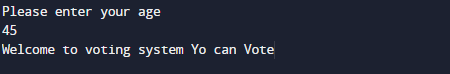Not valid condition.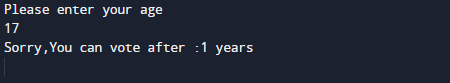In the above program, we have first declared and initialized a set variables required in the program.

• age= it will hold entered age by user
• shrt= it will hold value person short by how much.

After declaring variables in the next statement user will be prompted to enter a value and  which will be assigned to variable ‘age’.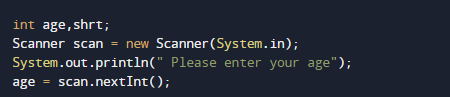And after that we calculate the value given age by user is valid for voting or not firstly check condition for valid voting if the given age is grater than 18 or equal to 18 ( age>=18) then it valid age.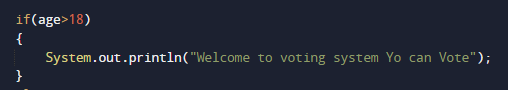Here what we have considered that age of given by user is eligible to vote if he attains age of 18 and more .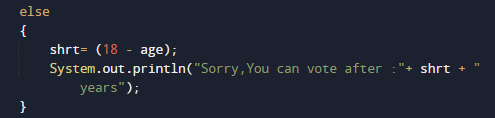If age of person is  not eligible to vote then we going to calculate the years after person can vote as shown in image above.

So in our program we calculate the age or person is eligible for vote or not.

In this tutorial we have learn about the Java program to find Voting Age Program in Java and its application with practical example. I hope you will like this tutorial.